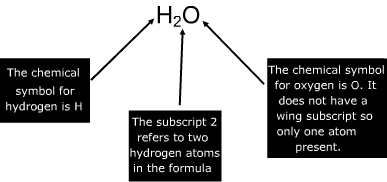Chemical Formula

Chemical Formula

A chemical formula shows the number and kinds of atoms present in a representative particle of a substance. For a molecule it would be known as a molecular formula, and for an ionic compound it would be referred to as a formula unit.Chemical Formula Questions:

1. Determine the number and types of atoms present in the chemical formula for glucose – C6H12O6.

Glucose has three different types of atoms present with varying amounts.

C is the chemical symbol for carbon which has 6 atoms present

H is the chemical symbol for hydrogen which has 12 atoms present

O is the chemical symbol for oxygen which has 6 atoms present.

2. Determine the number and types of atoms present in the chemical formula for calcium nitrate – Ca(NO3)2.

Calcium nitrate has three different types of atoms present with varying amounts. The parentheses around the NO3 signify that the number of atoms present in the parentheses must be multiplied by the subscript outside of the parentheses.

Ca is the chemical symbol for calcium which only has one atom present.

N is the chemical symbol for nitrogen which has (1 x 2) two atoms present.

O is the chemical symbol for oxygen which has (3 x 2) six atoms present.

 Related Links: Physical and Chemical Changes Quiz Chemical Equilibrium Quiz Chemical Equilibrium Quiz Chemical Energy Examples Acid vs. Base